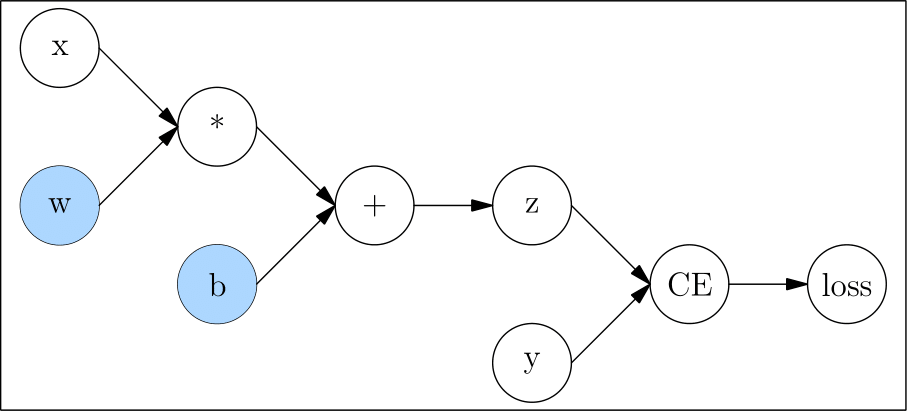OneFlow 提供了自动求导机制，可自动计算神经网络中参数的梯度。

## 计算图¶

import oneflow as flow

def loss(y_pred, y):
return flow.sum(1/2*(y_pred-y)**2)

x = flow.ones(1, 5)  # 输入
z = flow.matmul(x, w) + b

y = flow.zeros(1, 3)  # label
l = loss(z,y)## 自动求梯度¶

### backward 与梯度¶

l.backward()

tensor([[0.9397, 2.5428, 2.5377],
[0.9397, 2.5428, 2.5377],
[0.9397, 2.5428, 2.5377],
[0.9397, 2.5428, 2.5377],
[0.9397, 2.5428, 2.5377]], dtype=oneflow.float32)
tensor([[0.9397, 2.5428, 2.5377]], dtype=oneflow.float32)


### 对非叶子节点求梯度¶

from math import pi
n2 = flow.sin(n1)
n3 = flow.pow(n2, 2)

n3.backward()


tensor(-8.7423e-08, dtype=oneflow.float32)
tensor(2., dtype=oneflow.float32)


### 对一个计算图多次 backward()¶

n1 = flow.tensor(10., requires_grad=True)
n2 = flow.pow(n1, 2)
n2.backward()
n2.backward()


Maybe you try to backward through the node a second time. Specify retain_graph=True when calling .backward() or autograd.grad() the first time.

n1 = flow.tensor(10., requires_grad=True)
n2 = flow.pow(n1, 2)

n2.backward(retain_graph=True)
n2.backward()


tensor(20., dtype=oneflow.float32)
tensor(40., dtype=oneflow.float32)


n1 = flow.tensor(10., requires_grad=True)
n2 = flow.pow(n1, 2)

n2.backward(retain_graph=True)
n2.backward()


tensor(20., dtype=oneflow.float32)
tensor(20., dtype=oneflow.float32)


### 不记录某个 Tensor 的梯度¶

z = flow.matmul(x, w)+b

z = flow.matmul(x, w)+b


True
False

z_det = z.detach()


False


### 输出不是标量时如何求梯度¶

x = flow.randn(1, 2, requires_grad=True)
y = 3*x + 1
y.backward()


Check failed: IsScalarTensor(*outputs.at(i)) Grad can be implicitly created only for scalar outputs

x = flow.randn(1, 2, requires_grad=True)
y = 3*x + 1
y = y.sum()
y.backward()


tensor([[3., 3.]], dtype=oneflow.float32)


## 扩展阅读¶

x 张量中有两个元素，记作 $$x_1$$$$x_2$$y 张量中的两个元素记作 $$y_1$$$$y_2$$，并且两者的关系是：

$\mathbf{x} = [x_1, x_2]$
$\mathbf{y} = [y_1, y_2] = [3x_1+1, 3x_2+1]$

$\frac{\partial \mathbf{y}}{\partial \mathbf{x}} = \frac{[3x_1+1, 3x_2+1]}{[x_1, x_2]}$

$[\frac{\partial y_1}{\partial x_1}, \frac{\partial y_2}{\partial x_2}]$

$y = y_1 + y_2 = 3x_1 + 3x_2 + 2$

$\frac{\partial y}{\partial x_1} = \frac{\partial 3x_1 + 3x_2 + 2}{\partial x_1} = 3$
$\frac{\partial y}{\partial x_2} = \frac{\partial 3x_1 + 3x_2 + 2}{\partial x_2} = 3$

$J = \begin{pmatrix} \frac{\partial y_1}{\partial x_1} & \frac{\partial y_1}{\partial x_2}\\ \frac{\partial y_2}{\partial x_1} & \frac{\partial y_2}{\partial x_2} \end{pmatrix}\\ = \begin{pmatrix} \frac{\partial y_1}{\partial x_1} & 0 \\ 0 & \frac{\partial y_2}{\partial x_2} \end{pmatrix}$

$\begin{bmatrix} v_1\\ v_2 \end{bmatrix} \times \begin{pmatrix} \frac{\partial y_1}{\partial x_1} & 0 \\ 0 & \frac{\partial y_2}{\partial x_2} \end{pmatrix}= \begin{bmatrix} v_1 \frac{\partial y_1}{\partial x_1}\\ v_2 \frac{\partial y_2}{\partial x_2} \end{bmatrix}$

backward 方法是可以接受一个张量做参数的，该参数就是 VJP 中的 $$\mathbf{v}$$，理解以上道理后，还可以使用以下的方式对张量求梯度：

x = flow.randn(1, 2, requires_grad=True)
y = 3*x + 1
y.backward(flow.ones_like(y))

tensor([[3., 3.]], dtype=oneflow.float32)